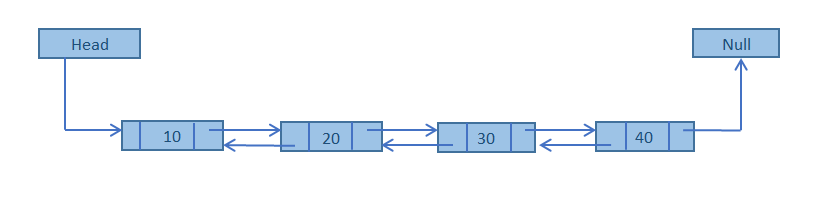Data Structure

In this type of liked list each node holds two-pointer field. Pointers exist between adjacent nodes in both directions.The list can be traversed either forward or backward.```NEXT holds the memory location of the Previous Node in the List.

PREV holds the memory location of the Next Node in the List.

DATA holds Data.

```

1.Doubly Linked List are more convenient than Singly Linked List since we maintain links for bi-directional traversing
2.We can traverse in both directions and display the contents in the whole List.
3.In Doubly Linked List we can traverse from Head to Tail as well as Tail to Head.
4. Each Node contains two fields, called Links , that are references to the previous and to the Next Node in the sequence of Nodes.
5. The previous link of the first node and the next link of the last node points to NULL.

### Function creates a simple doubly linked list

```/* Function creates a simple doubly linked list */

void createdub(node **start,node **end)

{

int i,item ,k=1;

printf("\nEnter number of node: ");

scanf("%d",&i);

while(i)

{   node *ptr;

printf("\nEnter the info for node %d : ",k);

scanf("%d",&item);

ptr=(node*)malloc(sizeof(node));

ptr->info=item;

if(*start==NULL)

{

ptr->prev = ptr->next = NULL ;

*start = *end = ptr ;

}

else

{

ptr->prev = *end;

(*end)->next = ptr ;

ptr->next= NULL;

(*end)=ptr;

}

i--;

k++;

}

}

```

### CREATING A SIMPLE DOUBLY LINKED LIST

```

/* CREATING A SIMPLE DOUBLY LINKED LIST */

#include<stdio.h>

#include<malloc.h>

#include<conio.h>

typedef struct Node

{

struct Node *prev;

int info ;

struct Node *next;

}node;

void createdub(node**,node**);

void display(node *);

void main()

{

int ch;

node *start ,*end ;

start = end = NULL;

clrscr();

createdub(&start,&end);

printf("\nThe list is : ");

display(start);

getch();

}

void createdub(node **start,node **end)

{

int i,item ,k=1;

printf("\nEnter number of node: ");

scanf("%d",&i);

while(i)

{   node *ptr;

printf("\nEnter the info for node %d : ",k);

scanf("%d",&item);

ptr=(node*)malloc(sizeof(node));

ptr->info=item;

if(*start==NULL)

{

ptr->prev = ptr->next = NULL ;

*start = *end = ptr ;

}

else

{

ptr->prev = *end;

(*end)->next = ptr ;

ptr->next= NULL;

(*end)=ptr;

}

i--;

k++;

}

}

void display(node *start)

{

while(start !=NULL)

{

printf("\t %d",start->info);

start = start->next;

}

}

```